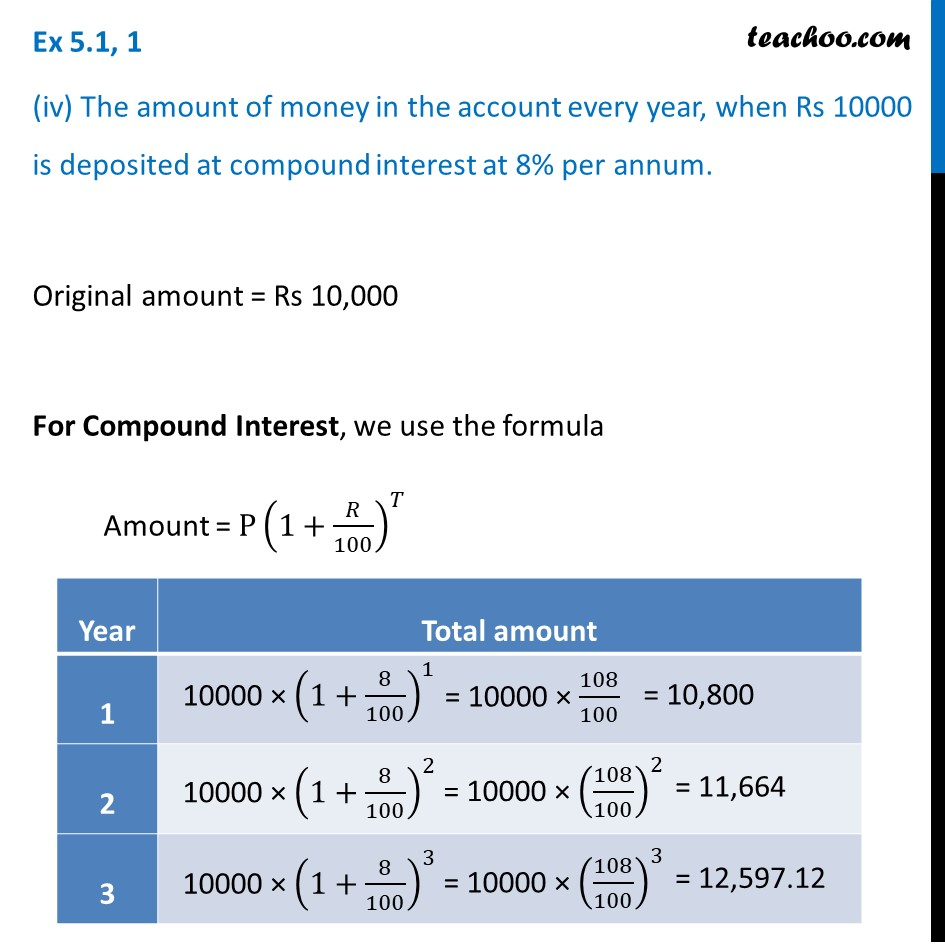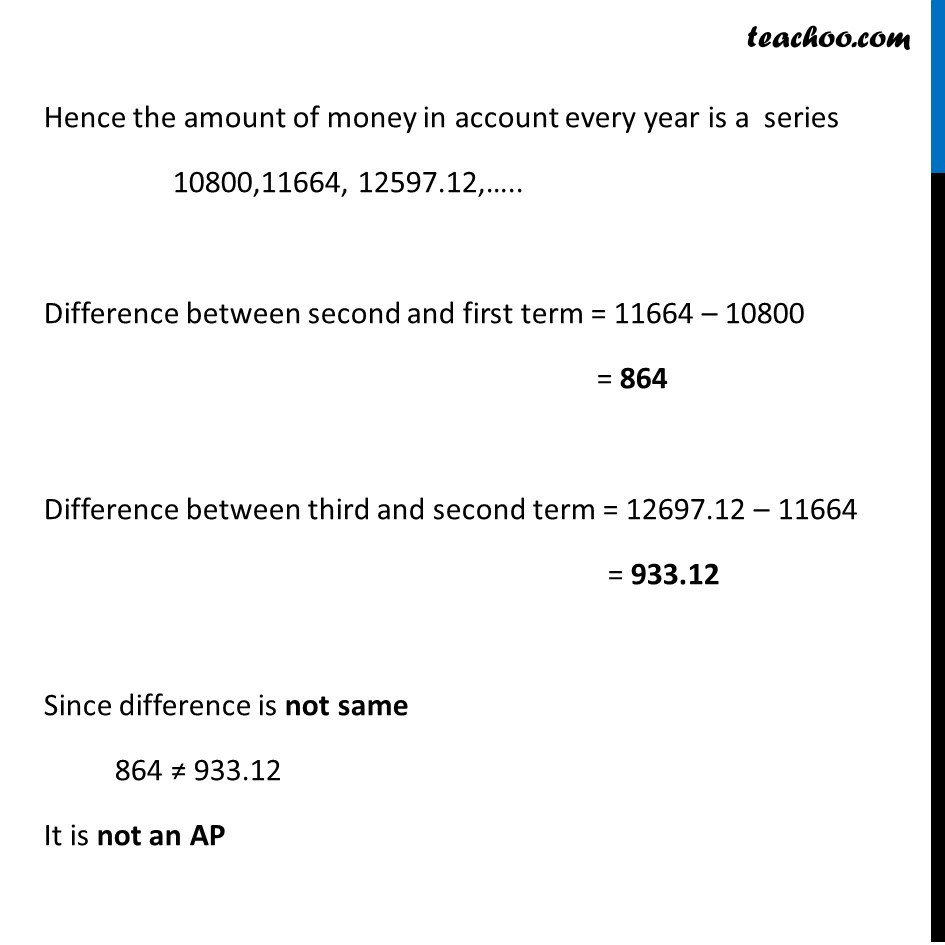1. Chapter 5 Class 10 Arithmetic Progressions (Term 2)
2. Serial order wise
3. Ex 5.1

Transcript

Ex 5.1, 1 (iv) The amount of money in the account every year, when Rs 10000 is deposited at compound interest at 8% per annum. Original amount = Rs 10,000 For Compound Interest, we use the formula Amount = P(1+𝑅/100)^𝑇 Hence the amount of money in account every year is a series 10800,11664, 12597.12,….. Difference between second and first term = 11664 – 10800 = 864 Difference between third and second term = 12697.12 – 11664 = 933.12 Since difference is not same 864 ≠ 933.12 It is not an AP